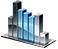Inheritance of classes

# Inheritance of classes

The NetTradeX language supports inheritance from a single base class. When your derived class inherits from a base class, it implements all the methods and properties of the base class, at the same time it may override methods of the base class.

When implementing a derived class, constructor calling the base class constructor may be needed, this can be accomplished by using the keyword ` super `. If the derived class does not call the base class constructor, the compiler will automatically call the default base class constructor.

Example of the class inheritance procedure:

` class Base { int a; Base(int a) { this.a=a; System.Print("Base conscructor is evoked"); } } class Derived: Base { int b; Derived(int a,int b) { super(a); this.b=b; System.Print("Derived conscructor is evoked"); } } int Run() { Derived x(5,10); System.Print("a="+x.a+" b="+x.b); return(0); } `

Output:

` Base constructor is evoked Derived constructor is evoked a=5 b=10 `
Converting an instance of a derived class object into an instance of the base class object can be done as follows:
` class Base { int a; Base(int a) { this.a=a; System.Print("Base conscructor is evoked"); } } class Derived: Base { int b; Derived(int a,int b) { super(a); this.b=b; System.Print("Derived conscructor is evoked"); } } int Run() { Derived x(11,22); Base @bObj=x; System.Print("bObj.a="+bObj.a); return(0); } `

The class can be specified with the keyword ` final ` before ` class `, in which case the class cannot be inherited. Also ` final ` can be specified before any class method that will lead to a ban of overriding this method in a derived class.

` final class A { } //This inheritance is forbidden //class B: A //{ //} class C { void D() final { } void E() { } } class F: C { // Can redefine the E method void E() { } //This override is prohibited //void D() //{ //} } int Run() { return(0); } `